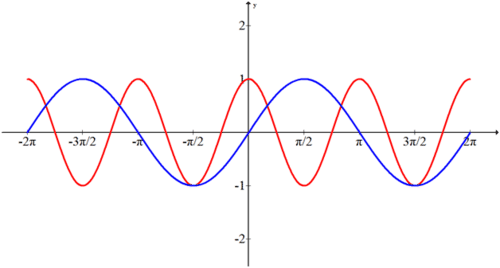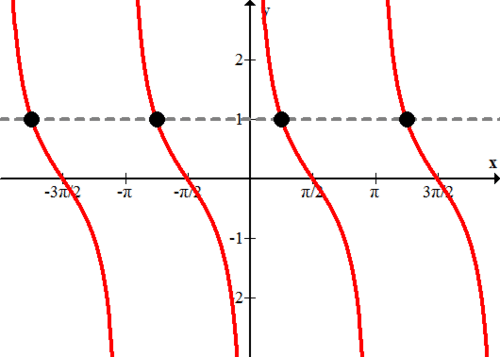6.5: Trigonometric Equations

•• Contributed by CK12
• CK12

Solving a trigonometric equation is just like solving a regular equation. You will use factoring and other algebraic techniques to get the variable on one side. The biggest difference with trigonometric equations is the opportunity for there to be an infinite number of solutions that must be described with a pattern. The equation $$\cos x=1$$ has many solutions including 0 and $$2 \pi .$$ How would you describe all of them?

Solving Trigonometric Equations

The identities you have learned are helpful in solving trigonometric equations.  The goal of solving an equation hasn’t changed.  Do whatever it takes to get the variable alone on one side of the equation.  Factoring, especially with the Pythagorean identity, is critical.

Try to give exact (non-rounded) answers when solving trigonometric equations. If you are working with a calculator, keep in mind that while some newer calculators can provide exact answers like $$\frac{\sqrt{3}}{2}$$ most calculators will produce a decimal of $$0.866 \ldots$$ If you see a decimal like $$0.866 \ldots,$$ try squaring it. The result might be a nice fraction like $$\frac{3}{4}$$. Then you can logically conclude that the original decimal must be the square root of $$\frac{3}{4}$$ or $$\frac{\sqrt{3}}{2}$$

When solving, if the two sides of the equation are always equal, then the equation is an identity. If the two sides of an equation are never equal, as with $$\sin x=3$$, then the equation has no solution.

Examples

Example 1

Earlier, you were asked how you could describe the many solutions of $$\cos x=1$$. When you type $$\cos ^{-1} 1$$ on your calculator, it will yield only one solution which is $$0 .$$ In order to describe all the solutions you must use logic and the graph to figure out that cosine also has a height of 1 at $$-2 \pi, 2 \pi,-4 \pi, 4 \pi \ldots$$ Luckily all these values are sequences in a clear pattern so you can describe them all in general with the following notation:

$$x=0 \pm n \cdot 2 \pi$$ where $$n$$ is an integer, or $$x=\pm n \cdot 2 \pi$$ where $$n$$ is an integer.

Example 2

Solve the following equation algebraically and confirm graphically on the interval $$[-2 \pi, 2 \pi]$$.

$$\cos 2 x=\sin x$$

\begin{aligned} \cos 2 x &=\sin x \\ 1-2 \sin ^{2} x &=\sin x \\ 0 &=2 \sin ^{2} x+\sin x-1 \\ 0 &=(2 \sin x-1)(\sin x+1) \end{aligned}

Solving the first part set equal to zero within the interval yields:

\begin{aligned} 0 &=2 \sin x-1 \\ \frac{1}{2} &=\sin x \\ x &=\frac{\pi}{6}, \frac{5 \pi}{6},-\frac{11 \pi}{6},-\frac{7 \pi}{6} \end{aligned}

Solving the second part set equal to zero yields:

\begin{aligned} 0 &=\sin x+1 \\-1 &=\sin x \\ x &=-\frac{\pi}{2}, \frac{3 \pi}{2} \end{aligned}

These are the six solutions that will appear as intersections of the two graphs $$f(x)=\cos 2 x$$ and $$g(x)=\sin x$$Example 3

Determine the general solution to the following equation.

$$\cot x-1=0$$

$$\cot x-1=0$$
$$\cot x=1$$

One solution is $$x=\frac{\pi}{4} .$$ However, since this question asks for the general solution, you need to find every possible solution. You have to know that cotangent has a period of $$\pi$$ which means if you add or subtract $$\pi$$ from $$\frac{\pi}{4}$$ then it will also yield a height of $$1 .$$ To capture all these other possible $$x$$ values you should use this notation.

$$x=\frac{\pi}{4} \pm n \cdot \pi$$ where $$n$$ is a integerNotice that trigonometric equations may have an infinite number of solutions that repeat in a certain pattern because they are periodic functions.  When you see these directions remember to find all the solutions by using notation like in this example.

Example 4

Solve the following equation.
$$4 \cos ^{2} x-1=3-4 \sin ^{2} x$$

\begin{aligned} 4 \cos ^{2} x-1 &=3-4 \sin ^{2} x \\ 4 \cos ^{2} x+4 \sin ^{2} x &=3+1 \\ 4\left(\cos ^{2} x+\sin ^{2} x\right) &=4 \\ 4 &=4 \end{aligned}

This equation is always true which means the right side is always equal to the left side.  This is an identity.

Example 5

Solve the following equation exactly.

$$2 \cos ^{2} x+3 \cos x-2=0$$

Note that $$\cos x \neq-2$$ which means only one equation needs to be solved for solutions.

\begin{aligned} 2 \cos x-1 &=0 \\ \cos x &=\frac{1}{2} \\ x &=\frac{\pi}{3},-\frac{\pi}{3} \end{aligned}

These are the solutions within the interval $$-\pi$$ to $$\pi .$$ since this represents one full period of cosine, the rest of the solutions are just multiples of $$2 \pi$$ added and subtracted to these two values.

$$x=\pm \frac{\pi}{3} \pm n \cdot 2 \pi$$ where $$n$$ is an integer

Review

Solve each equation on the interval $$[0,2 \pi)$$.

1. $$3 \cos ^{2} \frac{x}{2}=3$$

2. $$4 \sin ^{2} x=8 \sin ^{2} \frac{x}{2}$$

Find approximate solutions to each equation on the interval $$[0,2 \pi)$$

3. $$3 \cos ^{2} x+10 \cos x+2=0$$

4. $$\sin ^{2} x+3 \sin x=5$$

5. $$\cdot \tan ^{2} x+\tan x=3$$

6. $$\cot ^{2} x+5 \tan x+14=0$$

7. $$\sin ^{2} x+\cos ^{2} x=1$$

Solve each equation on the interval $$\left[0,360^{\circ}\right)$$.

8. $$2 \sin \left(x-\frac{\pi}{2}\right)=1$$

9. $$4 \cos (x-\pi)=4$$

Solve each equation on the interval $$[2 \pi, 4 \pi)$$

10. $$\cos ^{2} x+2 \cos x+1=0$$

11. $$3 \sin x=2 \cos ^{2} x$$

12. $$\tan x \sin ^{2} x=\tan x$$

13. $$\sin ^{2} x+1=2 \sin x$$

14. $$\sec ^{2} x=4$$

15. $$\sin ^{2} x-4=\cos ^{2} x-\cos 2 x-4$$